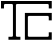## NIEM Progression 5

Disclaimer: This tool was developed by Tom Carlson Consulting LLC. It was created from the official distribution of the data model. Tom Carlson Consulting LLC makes neither claim nor warranty that this tool provides an accurate representation of the NIEM data model. For official and authoritative representations, please visit the official NIEM release website.

## cbrn:Equation

A mathematical equation and its coefficients.

cbrn:Equation holds other objects.

cbrn:Equation can hold any of these following objects, starting with the most specific and becoming more generic as you move down the list:

### cbrn:EquationCoefficientValueList

A list of values of the coefficients of an equation.

Holds numbers.

### cbrn:EquationCovarianceMatrixValueList

A white-space delimited list of values that provide the lower triangular half of an equation covariance matrix.

Holds numbers.

### cbrn:EquationKindCode

A kind of an equation.

Holds code table entries.

### cbrn:EquationDescriptionText

A free-form description of the equation type; it is intended for documentation purposes only. If the equation kind is Other, then this description shall be specified to explain the kind of equation. Polynomial equations shall report the linear term first as Term0 followed by the other terms. An example would be: Energy = Term0 + Term1*Ch + Term2*Ch**2

Holds text.

### cbrn:LowerLimitValue

A lowest value of X for which an equation is valid.

Holds numbers.

### cbrn:UpperLimitValue

A highest value of X for which an equation is valid.

Holds numbers.

### cbrn:RemarkText

A placeholder for comments intended to help the consumer of the data to understand better the information encapsulated by the parent element.

Holds text.